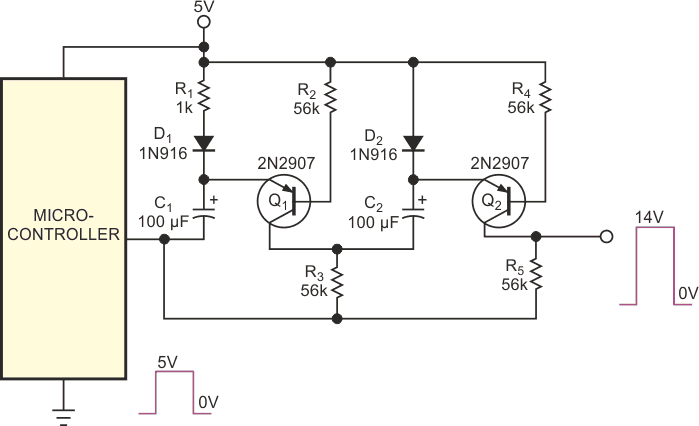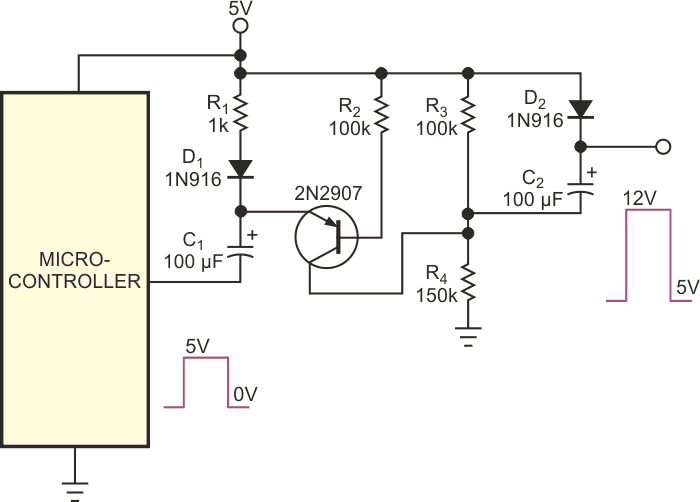# Circuit Forms Single-Pulse Voltage Multiplier

It is sometimes necessary for a microcontroller to generate a pulse whose voltage is higher than the power-supply voltage of the microcontroller. The circuit in Figure 1 allows you to generate 14 V pulses from a 5 V power supply. An adaptation of the circuit provides a 5 to 12 V programming pulse for programming the fuse bits in Atmel microcontrollers (Figure 2). The approach is economical, in that it combines the voltage-multiplier and pulse-amplifier functions. Moreover, the technique benefits from the absence of noise that would arise from a continuously running switching power supply.Figure 1. The simple circuit provides single pulses of 14 V from a 5 V power supply.

In Figure 1, when the microcontroller's output is low, C1 and C2 charge in parallel to nearly 5 . When the microcontroller switches to 5 V, it effectively makes the capacitors appear in series with the 5 V pulse, resulting in a pulse approaching three times the power-supply voltage at the output. C1 charges through D1, and R1 limits the charging current from the microcontroller's output to a few milliamperes. C2 charges through D2 and R3.Figure 2. This variation on the circuit in Figure 1 supplies fuse-programming pulses for Atmel AVR microcontrollers.

During the output pulse, C1 must supply the base current for Q1 and Q2, as well as the load current. Because the voltage drop across the diodes decreases as current through them diminishes, after a charging time of several time constants, the diode drop is only a couple of hundred millivolts. Therefore, pulses of nearly three times the power-supply voltage are possible. When the pulses are continuous or when they occur within a couple of time constants of power application, diode drops of approximately 1.5 V subtract from the output. Additional losses can arise from voltage drops across the resistance of the microcontroller and saturation losses in the transistors. You can reduce the saturation losses by reducing the values of R2 and R4, but be aware that reducing these values increases the droop rate of the output pulse. For some applications, you could omit D1 and replace D2 with a resistor, but the result would be longer charging and faster discharging for the capacitors. These trade-offs are acceptable for some applications.

The circuit in Figure 2 provides a 5 to 12 V programming pulse for Atmel AVR microcontrollers for the couple of hundred milliseconds the ICs require. Because the output during the time the microcontroller's output is low needs to be 5 V, you omit the second transistor and take the output directly from the cathode of D2. Because the output voltage needs to go to only 12 V, C2 charges from a voltage divider. C2 charges to only 2 V, which then appears in series with the 10 V from the series combination of C1 and the output of the microcontroller. You get no “free lunch” with this circuit. If the pulse initiation occurs before R3 and R4 sufficiently charge C2 (60 kΩ × 100 µF = 6 sec), the voltage is lower than intended. You can reduce the charge time of the circuit by reducing the values of the capacitors. C2 has the most effect because of the high-resistance charging path. However, reducing the capacitor values makes the output pulse droop more quickly.

EDN